# Indeterminate forms of Limits

Examples with detailed solutions and exercises that solves limits questions related to indeterminate forms such as :## Theorem

A second version of L'Hopital's rule allows us to replace the limit problem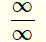with another simpler problem to solve.## Examples with Solutions

### Example 1

Find the limit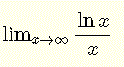Solution to Example 1:
Sinceand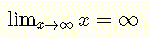we have the indeterminate formThe above L'Hopital's rule can be used to evaluate the given limit question as follows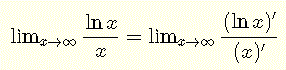Evaluate the derivatives in the numerator and the denominator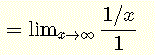Evaluate the limits in the numerator and denominatorWe now evaluate the given limit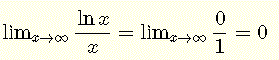### Example 2

Find $$\lim_{x\to \infty} x e^{-x}$$

Solution to Example 2:
Note that
$$\lim_{x\to \infty} x = \infty$$
and
$$\lim_{x\to \infty} e^{-x} = 0$$

This is the indeterminate form $$\infty \cdot 0$$. The idea is to convert it into to the indeterminate form $$\dfrac{\infty}{\infty}$$ and use L'Hopital's theorem. Note that
$$\lim_{x\to \infty} x e^{-x} = \lim_{x\to \infty} \dfrac{ x}{ e^x} = \dfrac{ \infty }{ \infty }$$

We apply the above L'Hopital's theorem
$$= \lim_{x\to \infty} \dfrac{ (x)'}{ (e^x)'} = \lim_{x\to \infty} \dfrac{ 1 }{ e^x} = 0$$

### Example 3

Find $$\lim_{x\to \infty} ( 1 + 1/x)^x$$

Solution to Example 3:
Note that $$\lim_{x\to \infty} (1 + 1/x) = 1$$ and the above limit is given by
$$\lim_{x\to \infty} ( 1 + 1/x)^x = 1^{\infty}$$

which is of the Indeterminate form $$1^{\infty}$$. If we let $$t = 1 / x$$ the above limit may written as
$$\lim_{x\to \infty} ( 1 + 1/x)^x = \lim_{t\to 0} ( 1 + t)^{1/t}$$ , note that as $$x\to \infty$$ , $$t\to 0$$

Let $$y = ( 1 + t)^{1/t}$$ and find the limit of $$\ln y$$ as t approaches 0
$$\ln y = \ln ( 1 + t)^{1/t} =(1 / t) \ln (1 + t)$$

The advantage of using $$\ln y$$ is that
$$\lim_{t\to 0} \ln y = \lim_{t\to 0} \dfrac {\ln (1 + t)}{t} = \dfrac{0}{0}$$

and the limit has the indeterminate form 0 / 0 and the first L'Hopital's rule can be applied
$$\lim_{t\to 0} \ln y = \lim_{t\to 0} \dfrac {ln (1 + t)}{t}$$
$$= \lim_{t\to 0} \dfrac{(ln (1 + t))'}{(t)'} = \lim_{t\to 0} \dfrac{1/(1+t)}{1} = 1$$

Since the limit of ln y = 1 the limit of y is e 1 = e, hence
$$\lim_{x\to \infty} ( 1 + 1/x)^x = e$$

### Example 4

Find the limit $$\lim_{x\to 0^+} ( 1 / x - 1 / \sin x )$$
Solution to Example 4:
Note that
$$\lim_{x\to 0^+} ( 1 / x ) = + \infty$$
and
$$\lim_{x\to 0^+} ( 1 / \sin x ) = + \infty$$

This limit has the indeterminate form $$\infty - \infty$$ and has to be converted to another form by combining $$1 / x - 1 / sin x$$
$$\lim_{x\to 0^+} ( 1 / x - 1 / \sin x ) = \lim_{x\to 0^+} \dfrac{\sin x - x}{x \sin x} = \dfrac{0}{0}$$

We now have the indeterminate form 0 / 0 and we can use the L'Hopital's theorem.
$$\lim_{x\to 0^+} \dfrac{\sin x - x}{x \sin x} = \lim_{x\to 0^+} \dfrac{(\sin x - x)'}{(x \sin x)'} = \lim_{x\to 0^+} \dfrac{\cos x - 1}{ \sin x + x \cos x} = \dfrac{0}{0}$$

We have again the indeterminate form 0 / 0 and use the L'Hopital's theorem one more time.
$$= \lim_{x\to 0^+} \dfrac{(\cos x - 1)'}{ (\sin x + x \cos x)'}$$
$$= \lim_{x\to 0^+} \dfrac{-\sin x}{ \cos x + \cos x - x \sin x } = \dfrac{0}{2} = 0$$

### Example 5

Find the limit $$\lim_{x\to 0^+} x^x$$
Solution to Example 5:
We have the indeterminate form 00. Let y = x x and ln y = ln (x x) = x ln x. Let us now find the limit of ln y
$$\lim_{x\to 0^+} \ln y = \lim_{x\to 0^+} x \ln x = 0 \cdot \infty$$

The above limit has the indeterminate form $$0 \cdot \infty$$. We have convert it as follows
$$\lim_{x\to 0^+} x \ln x = \lim_{x\to 0^+} \dfrac{\ln x}{1/x} = \dfrac{\infty}{\infty}$$

It now has the indeterminate form $$\dfrac{\infty}{\infty}$$ and we can use the L'Hopital's theorem
$$\lim_{x\to 0^+} \dfrac{\ln x}{1/x} = \lim_{x\to 0^+} \dfrac{(\ln x)'}{(1/x)'}$$
$$= \lim_{x\to 0^+} \dfrac{1/x}{-1/x^2}$$
$$= \lim_{x\to 0^+} - x = 0$$

The limit of ln y = 0 and the limit of y = xx is equal to
$$e^0 = 1$$

## Exercises

Find the limits
1. $$\lim_{x\to \infty} (\ln x)^{1/x}$$
2. $$\lim_{x\to \infty} (\ln x - \ln (1 + x))$$
3. $$\lim_{x\to \infty} \dfrac{x}{e^x}$$
4. $$\lim_{x\to 0^+} x^{\sin x}$$

1. 1
2. 0
3. 0
4. 1